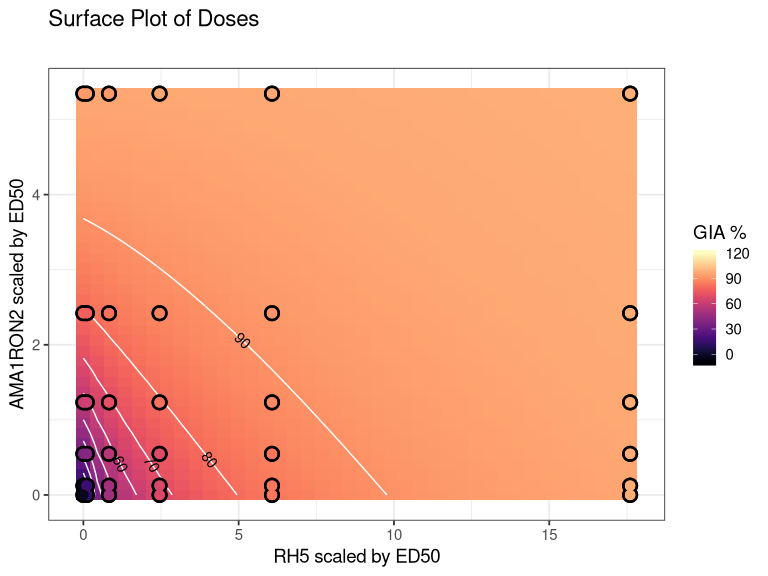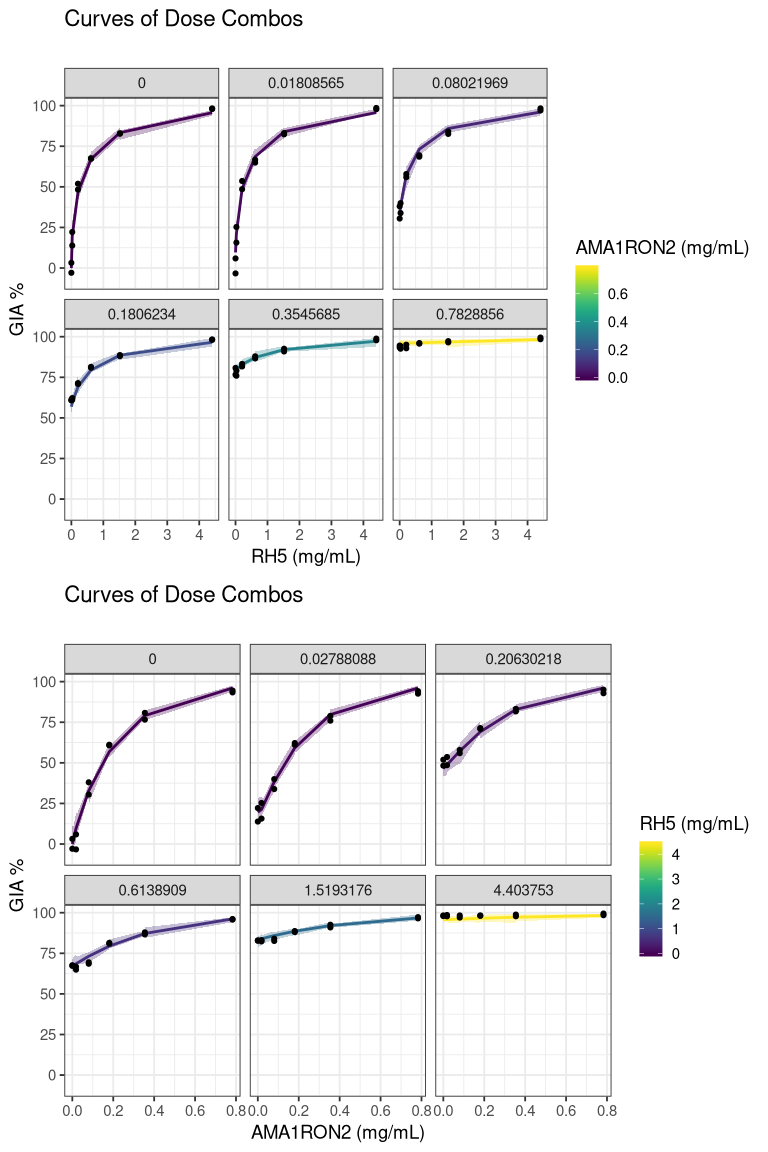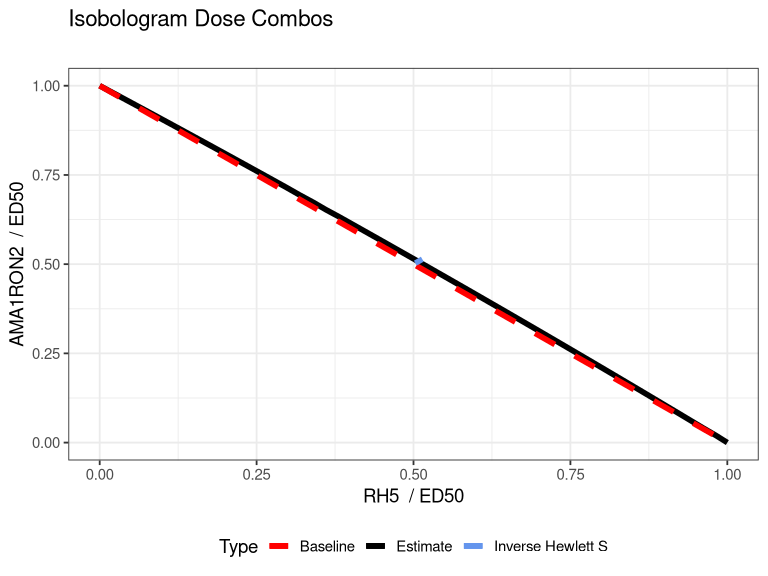# Installation

To install loewesadditivity, run the following code.

if(!require(loewesadditivity)){
library(devtools)
}
library(loewesadditivity)

# Quickstart

library(ggplot2)
library(dplyr)
library(knitr)
library(kableExtra)

results <- rh5_ama1ron2 %>%
rename(dose_A = "RH5", dose_B = "AMA1RON2")  %>%
fortify_gia_data() %>%
estimate_params()

resultsparams_est #> param mean lower upper #> 1 beta_A 0.25084255 0.2178025 0.290732958 #> 2 beta_B 0.14635802 0.1341838 0.158116394 #> 3 gamma_A 0.52662383 0.4780797 0.573632561 #> 4 gamma_B 0.91952943 0.8296499 1.028295864 #> 5 tau_1 -0.05780865 -0.1239247 -0.006908983 #> 6 tau_2 0.10477611 -5.2028784 14.953877207 ## Look at the raw data This is a raw set of GIA data that has been collected and uploaded to this package. We measure the GIA% for different experiments, plates (a or b) and repetitions. We are using the compounds AMA1RON2 and RH5. The variables iRBC and uRBC are optional rows which measure the minimal and maximum GIA respectively. data(rh5_ama1ron2) rh5_ama1ron2 %>% head() %>% knitr::kable() %>% kableExtra::kable_styling(full_width = T, font_size = 7) well AMA1RON2 RH5 exp1arep1 exp1arep2 exp1arep3 exp1brep1 exp1brep2 exp1brep3 exp2arep1 exp2arep2 exp2arep3 exp2brep1 exp2brep2 exp2brep3 iRBC NA NA 0.448 0.462 0.447 0.456 0.465 0.447 0.450 0.453 0.45 0.470 0.444 0.423 uRBC NA NA 0.063 0.063 0.061 0.066 0.064 0.063 0.073 0.071 0.07 0.068 0.068 0.068 RPMI 0.0000000 0 0.440 0.438 NA NA NA NA 0.453 0.472 NA NA NA NA comb 0.0180857 0 0.427 0.430 NA NA NA NA 0.460 0.468 NA NA NA NA comb 0.0802197 0 0.328 0.340 NA NA NA NA 0.291 0.319 NA NA NA NA comb 0.1806234 0 0.214 0.218 NA NA NA NA 0.212 0.223 NA NA NA NA ## Fortify data The standard output we use is that of the long, data.frame with the columns 1. dose_A 2. dose_B 3. GIA where dose_A and dose_B are the unscaled doses (e.g. mg/mL) and GIA is the percent GIA. We provide the function fortify_gia_data() which works when the data is in the above format with column headings of exp{x}{y}rep{z} along with the two doses (must have names dose_A and dose_B.  rh5_ama1ron2_f <- rh5_ama1ron2 %>% rename(dose_A = 'RH5', dose_B = 'AMA1RON2') %>% fortify_gia_data() rh5_ama1ron2_f %>% head() %>% kable(align = "c") %>% kableExtra::kable_styling(full_width = F) well dose_A dose_B uRBC iRBC exp_num plate GIA comb 0 0.0180857 0.0665 0.45125 1 a 5.91293 comb 0 0.0180857 0.0665 0.45125 2 a -3.31384 comb 0 0.0802197 0.0665 0.45125 1 a 30.47433 comb 0 0.0802197 0.0665 0.45125 2 a 38.01170 comb 0 0.1806234 0.0665 0.45125 1 a 61.14360 comb 0 0.1806234 0.0665 0.45125 2 a 60.75374 The fortification has averaged over the repetitions but has kept the plates and the experiments. ## Estimate parameters You can use the function estimate_params() to estimate the parameters. The parameters and their meaning can be found in the documentation of ?base_GIA. ## Set up initial guesses and parameters model_params <- c( "beta_A" = .5, "beta_B" = .5, "gamma_A" = .5, "gamma_B" = .5, "tau_1" = 0, "tau_2" = 0 ) n_boot <- 10 fn_list <- NULL alpha <- .05 verbose <- TRUE out <- estimate_params( data = rh5_ama1ron2_f, init_params = model_params, n_boot = n_boot, alpha = alpha, verbose = verbose ) #>  "Estimating best parameters" #>  "Starting the bootstrap" #>  "Generating parametric error" Hewlett’s S can be found below. outS_est  %>%  kable(align = "c", digits = 3) %>%
kableExtra::kable_styling(full_width = F)
stat mean lower upper
S 0.985 0.973 0.994

The parameter estimates from the model are shown here:

outparams_est %>% kable(align = "c", digits = 3) %>% kableExtra::kable_styling(full_width = F) param mean lower upper beta_A 0.250 0.226 0.268 beta_B 0.146 0.140 0.153 gamma_A 0.527 0.496 0.553 gamma_B 0.921 0.835 0.963 tau_1 -0.058 -0.120 -0.038 tau_2 0.288 -5.018 3.390 Finally, the (first six) individual observation estimates and 95% CIs can be found here: outGIA_est %>% head()  %>%  kable(align = "c", digits = 3) %>%
kableExtra::kable_styling(full_width = F)
well dose_A dose_B uRBC iRBC exp_num plate GIA mean lower upper
comb 0 0.018 0.066 0.451 1 a 5.913 9.605 1.501 19.086
comb 0 0.018 0.066 0.451 2 a -3.314 9.605 3.411 16.810
comb 0 0.080 0.066 0.451 1 a 30.474 32.844 30.438 36.761
comb 0 0.080 0.066 0.451 2 a 38.012 32.844 24.977 34.296
comb 0 0.181 0.066 0.451 1 a 61.144 56.863 55.289 62.165
comb 0 0.181 0.066 0.451 2 a 60.754 56.863 53.508 60.260

## Plot results

We can plot the estimated surface, individual curves, and the isobologram.

# Note xlab is dose_A and ylab is dose_B
g1 <- plot_surface(out, xlab = "RH5", ylab = "AMA1RON2")g2 <- plot_curves(out, dose_A = "RH5", dose_B = "AMA1RON2" )g3 <- plot_isobologram(out, dose_A = "RH5", dose_B = "AMA1RON2" )## Simulation of coverage

We provide the function simulate_coverage() as a way to get an estimate of the power of the experiment to detect synergy or antagonism. For given model parameters, an experimental grid of dose combinations, and an assumed noise structure for GIA, we can determine 1) percent of times we expect 0 to in the 95% CI of the interaction parameter $$\tau_1$$ and 2) percent of times we expect the true given value of the model parameters to be in the 95% CI.

WARNING It is advised to use at least 100 bootstraps for each of 10 simulations but is not shown here.

  model_params <- c("beta_A" = .250, "beta_B" = .146,
"gamma_A" = .527, "gamma_B" = .921,
"tau_1" = -.058, "tau_2" = -.289)
experimental_grid <- make_grid(par = model_params,
n = 5)
n_boot <- 3
n_sims <- 5
GIA_fn <- base_GIA
S_fn <- calc_S_base
fn_list <- NULL
alpha <- .05
verbose <- TRUE
out <- simulate_coverage(n_sims = n_sims,
n_boot = n_boot,
verbose = FALSE,
experimental_grid = experimental_grid,
model_par = model_params,
alpha = .05,
noise_par = c("a0" = 3, "a1" = .01),
GIA_fn = base_GIA,
fn_list = NULL)

out
#> $interaction_cov #>  60 #> #>$params_cov
#>  beta_A  beta_B gamma_A gamma_B   tau_1   tau_2
#>      60      20      20      40      40      60
#>
#> $pos_tau #>  0 #> #>$neg_tau
#>  40

## Design an experiment

A unique feature of loewesadditivity is the ability to generate code to use to create your own coverage simulation in conjunction with a (hopefully) intuitive shiny App. Run the below code, copy and paste the results into R and see what happens!

Ex.

 out <- design_experiment(n_rep = 2)
#>
#> levels_A <- c(0, 0.0625, 0.125, 0.25, 0.5, 1, 2, 4)
#> levels_B <- c(0, 0.125, 0.25, 0.5, 1, 2, 4, 8)
#> par <- c(  'beta_A' = 1,
#>   'beta_B' = 2,
#>   'gamma_A' = 0.5,
#>   'gamma_B' = 0.5,
#>   'tau_1' = 3,
#>   'tau_2' = 0.05)
#> my_grid <- design_grid(levels_A = levels_A,
#>   levels_B = levels_B,
#>   n_rep = 2)
#> ## SIMULATE COVERAGE
#> sim_results <- simulate_coverage(n_sims = 100,
#>   n_boot = 100,
#>   experimental_grid = my_grid,
#>   model_par = par,
#>   alpha = .05,
#>   noise_par = c('a0' = 3,
#>   'a1' = 0.01))
#> ## LOOK AT RESULTS
#> sim_results
#> ## Uncomment below to write the grid to a .csv file you can open in Excel or google spreadsheets
#> #write.csv(sim_results, 'coverage_results.csv')